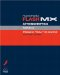# BUILDING EXPRESSIONS

 An expression is a phrase or a collection of variables, numbers, text, and operators that evaluates to a value. To understand this, take a look at the following example: ` oneDozen = 6 * 2; ` To the right of the equals sign, you see 6 * 2: This is an expression. When this line of ActionScript is executed, the expression 6 * 2 is replaced by the result of 6 multiplied by 2, or 12 . Thus, oneDozen equals 12. In addition to the already-mentioned scripting elements, an expression can contain variables, arrays, even function calls anything that when evaluated returns a value. For example: ` total = subTotal + tax; ` Here, the value of total is based on the result of adding the variable subTotal to the variable tax ; subTotal + tax is the expression. ` discount = totalPrice * employeeDiscount; ` Here, the value of discount is based on the result of multiplying the variable totalPrice by the value of the third element in the employeeDiscount array; totalPrice * employeeDiscount is the expression. ` usDollars = 10; japaneseYen = convertToYen(usDollars); ` Here, the value of japaneseYen is based on the value returned by a call to the convertToYen() function making the function call itself the expression. NOTE For more information about functions, see Lesson 5, Using Functions. Expressions are used to: Set variable values Set array-element values Determine whether conditions are being met (using the comparison operators) Dynamically name movie clips, variables, and objects Call functions dynamically More By using expressions, you can avoid hard-coding values that will remain the same no matter what. And by assigning and manipulating values via expressions, you can make the data used by your scripts dynamic resulting in more interactive projects. You'll find that many of the scripts in this book rely on expressions that's because without them, your project plays back in exactly the same way each time it's viewed.Macromedia Flash MX ActionScripting: Advanced Training from the Source
ISBN: 0201770229
EAN: 2147483647
Year: 2002
Pages: 161